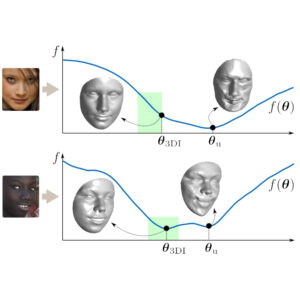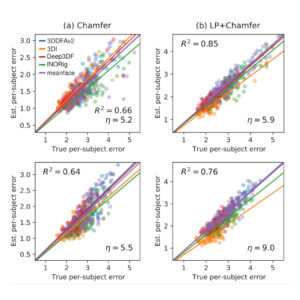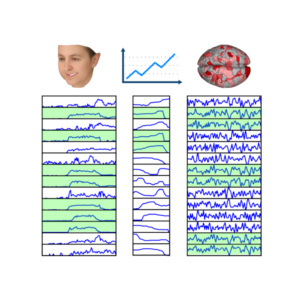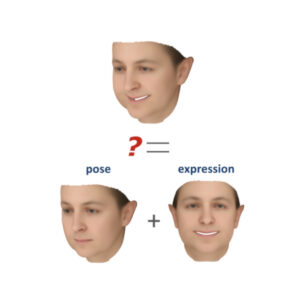•We present the CUDA code of our optimization-based 3DMM fitting method (i.e., no learning), which was first presented in ECCV’20. The journal version of the paper (currently under revision) presented a significant speed up with a new optimization approach, making the method feasible for real-life applications. 3DI is an optimization-based 3DMM fitting (3D reconstruction) method that enforces inequality constraints on 3DMM parameters and landmarks, thus significantly restricts the search space and rules out implausible solutions (Figure 1). 3DI is not a…

•Our IJCB’23 study exposed a significant problem with the benchmark procedures of 3D face reconstruction — something that should make any researcher in the field worry. That is, we showed that the standard metric for evaluating 3D face reconstruction methods, namely geometric error via Chamfer (i.e., nearest-neighbor) correspondence, is highly problematic. Results showed that the Chamfer error does not only significantly underestimate the true error, but it does so inconsistently across reconstruction methods, thus the ranking between methods can be artificially…

•In CVPR’20 presented a new method (with code) for finding the largest subset of synchronized time series from a given set of time series. Specifically, we aim to find the largest subset of time series such that all pairs of in the subset are correlated at least by a (given) threshold value $\rho$. This is an NP-hard problem and the exact solution is, in general, unfeasible. We propose a new method, called SyncRef, for finding an approximate solution in an efficient…

•This study on facial analysis pipelines shows the limitations of using a Weak Perspective (WP) camera when it comes to decoupling pose and expression from face videos. That is, when decoupling of facial pose and expression within images requires a camera model for 3D-to-2D mapping when done through 3D reconstruction. The weak perspective (WP) camera has been the most popular choice; it is the default, if not the only option, in state-of-the-art facial analysis methods and software. WP camera is justified…

• Some matrix norms are significantly costlier than others. For example, the matrix-2 norm requires computation of eigenvalues, whereas the matrix-1 norm is simply computed by finding the column of the matrix with the largest (absolute) sum (p283 of Carl D. Meyer). Thus, the following question becomes relevant: Can we use some matrix norm in place of some other norm? Fortunately, for some applications, we can do this. First of all, for analyzing limiting behavior, it makes no difference whether we use…

• Well, it depends on how you compute it. If you compute it by using the definition of determinant directly, in fact it can take more than 10¹⁴¹ years, as we’ll see.${}^\dagger$ Let’s recall the definition first. The determinant of a matrix $\mathbf A$ is $$\text{det}(\mathbf A) = \sum\limits_p \sigma(p) a_{1p_1} a_{2p_2} \dots a_{np_n},$$ where the sum is taken over the $n!$ permutations $p=(p_1,p_2,\dots,p_n)$ of the numbers $1,2,\dots,n$. The total number of multiplications in this definition are $n! (n-1)$. Based on my…

• Reflectors are a class of matrices that are not introduced in all linear algebra textbooks. However, the book of Carl D. Meyer uses this class of matrices heavily for various fundamental results. Indeed, the book uses reflectors for theoretical reasons, such as proving the existence of fundamental transformations like SVD, QR, Triangulation, or Hessenberg decompositions (more to come below), as well as applications, such as the implementation of the QR algorithm via the Householder transformation or solution of large-scale linear systems…

• These three inequalities often tend to appear as a package in many textbooks about real analysis, signal processing or linear algebra. It is good to know the main reason that we see this package all the time, and to separate the role of each of these three fundamental inequalities. The overall reason is that, these inequalities are the key to generalize the facts about vectors in 2D/3D spaces and the Euclidean norm to higher dimensional spaces and (non-Euclidean) $p$-norms. Each of…

• The triangle inequality $$||x+y||\leq ||x|| + ||y||$$ is possibly the earliest inequality that we learn. Starting from high school, we learn that the shortest distance between two points is a single straight line, and if we want to travel the same distance by two or more straight lines, we will travel longer. We know very well that the triangle inequality that we so intuitively get is not limited only to the 2D or 3D spaces that we can visualize, but to…

• The four fundamental spaces of a matrix $A$, namely the range and the nullspace of itself $A$ or its transpose $A^T$, are the heart of linear algebra. We often find ourselves in need of computing a basis for the range or the nullspace of a matrix, for theoretical or applicational purposes. There are many ways of computing a basis for the range or nullspace for $A$ or $A^T$. Some are better for application, either due to their robustness against floating point…